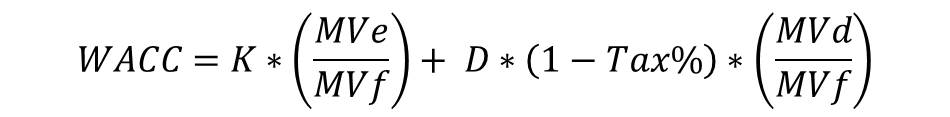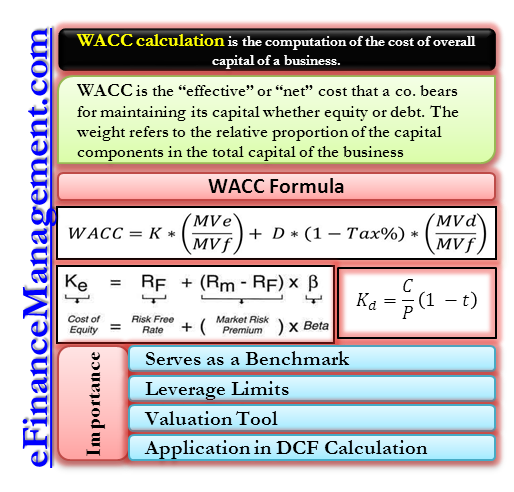# WACC Calculation

WACC calculation is the computation of the cost of overall capital of a business. The capital structure of a business comprises of components of debt and equity which have been procured at different cost. The calculation of WACC gives an aggregated and an all inclusive cost that is computed after taking into account the varying cost structure of all the capital components.

## What is WACC?

WACC or Weighted Average Cost of Capital is the “effective” or “net” cost that a business bears for maintaining its capital whether equity or debt. The weight refers to the relative proportion of the capital components in the total capital of the business. The cost of total funds of a business cannot be known by studying the capital components in isolation. The true picture emerges when they are studied collectively under a single lens. That is exactly the purpose of a WACC calculation. After all, the value of any enterprise is the combined effect of both equity and debt on its balance sheet. WACC calculation aggregates the various sources of funds. This provides a singular yet all-encompassing number to judge the cost-effectiveness.

## WACC Calculation

### WACC FormulaOr the extended formula looks like this:

WACC =Cost of Equity * % of Equity+ Cost of Debt(1-t) * % of Debt+ Cost of Preferred Stock * % of Preferred Stock

### Breaking down the Formula

To appreciate the WACC calculation in its entirety it helps to understand the derivation and rationale behind its components.

#### Cost of Equity

The cost of equity is generally derived using the CAPM model that lays out the cost of equity as follows:

Ke = Rf + β (Rm-Rf)
Rf = Risk-free Rate. In economic terms, it is the rate at which an investor can earn returns without taking any risk. The US treasury bill rate can be considered as a risk-free rate.
β = Beta factor. It is the sensitivity index of an asset. It indicates the magnitude of movement in the underlying asset when the market moves by 1.
Rm = Market Rate of Return. Denotes the returned earned by the basket of stocks comprising the market as a whole. The Rm may also be industry specific like the real estate index or the utility index. It can also be a collective index such as the S&P 500.

#### Cost of Debt

Kd = Interest (1-t)/Value of Debt
Interest: This formula is very intuitive. It occurs naturally that the cost of debt will be the interest one pays for it. However, what is to be understood is the (1-t) factor.
Interest on borrowings is basically tax deductible. This means that interest leads to prevention of outflow of cash in the form of taxes. Therefore, the effective cost of debt is less by the proportion of tax component.

#### Cost of Preference share

Kp = D0/P0

D0= Dividend (generally at a fixed rate); P0= Price of preference share.

Like interest payments are a fixed obligation for debt finance, dividends are a fixed obligation for preference share capital. Since dividends are paid out of after-tax profits, they do not entail any tax shield benefits.

## WACC Calculation with Practical Example

The Capital Structure of a company is as under

 Debentures (\$100 per debenture) \$500,000 Preference Share (\$100 per share) \$500,000 Equity Shares (\$10 per Share) \$1,000,000 \$2,000,000

The Market prices of the securities are as under
Debentures \$105 per Debenture
Preference Shares \$110 per Preference Share
Equity Share \$ 24 each

(1) \$ 100 per debenture, redeemable at par, 10 % coupon rate, applicable tax rate is 35%
(2) \$100 preference share, currently trading at \$ 110, 12% coupon rate.
(3) The prevailing risk-free rate on T-Bills is 5.5%. The Average market risk premium is 8%. The beta of the company is 1.8

Carrying out the WACC calculation using market value weights

Cost of Debentures
= Kd = Interest (1-t)/Value of Debt
= 10 (1-35%)/100 = 6.5%

Cost of Preference Shares
= Kp = Dividend Per Share/ Market Price Per Share
= \$12/\$110 = 10.9%

Cost of Equity Shares
= Ke = Rf + β (Rm -Rf)
= 0.055 + 1.8 (0.08-0.055)
=10 %

WACC of Capital Structure Using Market Value Weights

 Source of Funds Market Value of Capital (A) Weight (A/B) =C After Tax Cost of Capital (D) WACC(C*D) =E 10 % Debentures \$525,000 0.151 6.50% 0.98% 12% Preference Share Capital \$550,000 0.158 10.90% 1.72% Equity Share Capital \$2,400,000 0.691 10% 6.91% (B) \$3,475,000 9.61%

From the above WACC calculations, the net or effective cost that the business bears on its capital structure as a whole is 9.61%

Working Notes

Computation of Market Values

10% Debentures = (\$500,000/100) * \$105
= \$525,000

12% Preference Shares = (\$500,000/100) * \$110
=\$550,000

Equity Shares = (\$1,000,000/10) * \$24
=\$2,400,000

## Why is WACC Calculation Important?### Serves as a Benchmark

WACC gives the overall cost of capital. This information is necessary to have when analyzing prospective projects and investments. WACC is the minimum return as a business should be able to generate to break even. If a project ROI is less than the WACC, the business shall end up losing money. WACC is, therefore, an important benchmark against which all future investments must be compared.

### Leverage Limits

Using a WACC calculation the management is instantly able to analyze the impact of leverage on its capital structure. The WACC combines the cost of both equity and debt to provide an aggregated number. Using advanced models for WACC calculation the management can also arrive at the exact point at which the cost of additional debt outweighs its benefits. Therefore, the decisions regarding the quantum and cost of debt to be taken on can be made easily using simple WACC calculation.

### Valuation Tool

WACC is also an effective valuation tool for the business of companies. Analysts particularly perform WACC calculations to arrive at the combined rate that is used to value the firm. WACC is a fundamental and a simple check to ensure whether a company satisfies the preliminary parameters to qualify as an investable business. For example, a higher proportion of debt increases the risk factor since the company is exposed to liquidity risks. This, in turn, caused the investors to demand a higher rate of return. Therefore, the overall WACC goes up leading to a fall in valuation.

### Application in DCF Calculation

WACC is also used as the discount rate in NPV computation. Since WACC is an all-inclusive rate it constitutes the most suitable rate for discounting cash flows that occur to the entity as a whole.1

1.
WACC (Weighted Average Cost of Capital): WACC Formula and Real Examples. Wall Street Prep. December 2018. [Source]
Last updated on : December 31st, 2018